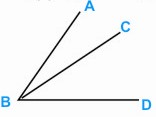HOME MATH DICTIONARY DOWNLOAD FEEDBACK DISCLAIMER
 Question: What is Bisect or a Bisector ? Answer: An angle is bisected when it is cut into two equal parts. In geometry, bisection is the division of something into two equal parts, usually by a line, which is then called a bisector. The most often considered types of bisectors are segment bisectors and angle bisectors. An angle bisector divides the angle into two equal angles. An angle only has one bisector. Each point of an angle bisector is equidistant from the sides of the angle. The interior bisector of an angle is the line or line segment that divides it into two equal angles on the same side as the angle.For e.g. the angle ABD is bisected by the line BC. BC is called the bisector.